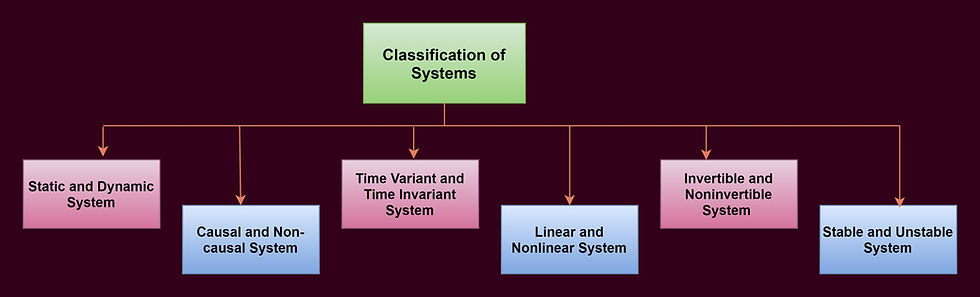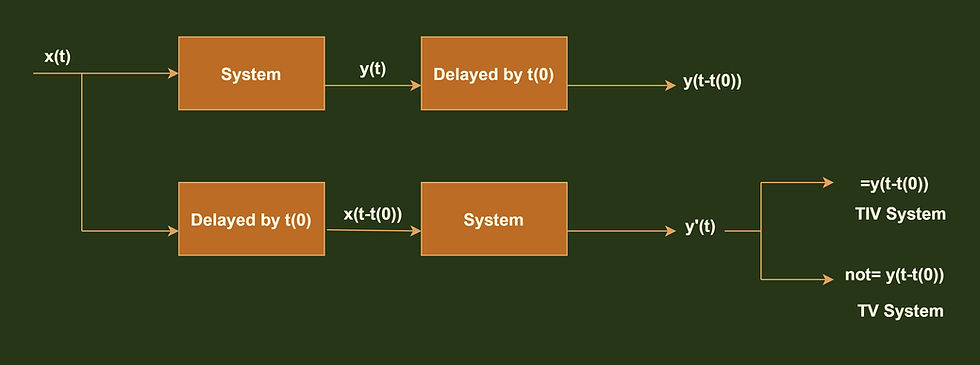top of page

# What does System signifies-In Signals and Systems

Updated: Aug 10

The meaningful interconnection between a physical device and component is called a system. A #system can also be defined as an operator that maps the relationship between the input signal and the output signal by the process of transformation. The article overlays an absolute overview of the system and its classification.System Representation

Alike to #signal, the system can also be broadly classified into a continuous-time system and discrete-time system. In continuous-time, both the input and outputs are continuous-time signals whereas in a discrete-time system both the input and outputs are discrete-time signals. The example of a system is the electrical system, mechanical system, etc.Continuous and Discrete time system

Classification of systemsSystem Classification

1) Static and Dynamic system

In a static system, the output at any instant of time depends only on the present value of the input. This signifies that the output does not depend on the past or future values of the input, therefore a static system is also called a system with no memory or energy storage elements. Let's understand the static system with the help of an example.

`Example:- y(t)=2x(t)`

Note:- Here '2' is the coefficient, which does not affect the system.

for the above example substitute different values of t and check the system performance.

let us check for t= -1,0,1

for t=-1; y(-1)=2x(-1) ==> the output depends on the present value of input.

for t=0; y(0)=2x(0) ==> the output depends on the present value of input.

for t=1; y(1)=2x(1) ==> the output depends on the present value of input.

Hence the system is static.

In a dynamic system, the output at any instant of time depends on the past or future values of input. Therefore dynamic systems are called systems having memory. let's check for the dynamic system the same as we did for the static one.

```Example:- y(t)= x(t)+x(t+1)+x(t-1).

```

For the above example to check if it is a static or dynamic system substitute different values of t.

let's check for t=0,1

for t=0; y(0)= x(0)+x(0+1)+x(0-1).

y(0)= x(0)[present value]+x(1)[future value]+x(-1)[past value]

Here for t=0; the output depends on future as well as past values of input with respect to zero which clearly indicates that the system is a dynamic system. Hence no need to check for other values.

2) Causal and Non-causal system.

The response of a casual system to input does not depend on future values of that input but depends only on the present or the past values of the input. Whereas if the response of the system to an input depends on the future value of that particular input then the system is a non-causal system. Let us understand the concept with the help of some examples.

Examples:-

1. y(t)= x(t) ==> casual system(check by substitution of different values of t ).

2. y(t)=x(t-1) ==> causal system.

3. y(t)=x(t+2) ==> non-causal system.

4. y(t)=x(t)+x(t+1)+x(t-1) ==> non-causal system.

Additional point:- The Anti-causal system is a system in which the output of the system depends only on the future values of input.

3) Time-variant and Time-invariant system.

This is a very essential type of classification of systems. A time-invariant system is one whose input-output relation do no vary with time, in other words, a time-invariant system is a system in which any time delay in the input must be reflected in the output. A system that does not follow this property is called a time-variant system.A pictorial representation to identify TIV and TV System

Let us understand the concept of Time-variant and Time-invariant system with some examples as some times it becomes complex to identify the system.

Sample:-

• y(t)=x(2t)

here x(t) ---> system ---> after #scaling by a factor of 2 ---> x(2t) = y(t)

Delayed output

```y(t) --> y[t-t(0)] = x[2(t- t(0))]   y(t) is delayed by t(0)
y(t)= x(2t-2t(0))```

Delayed input

```x(t) --> x(t- t(0)) =x(2t-t(0))    x(t) is delayed by t(0)
x(t)=x(2t-t(0))```

The input and output are no same after the #delay operation,hence the system is a Time-variant system.

• y(t)= 2+ x(t)

here x(t) ---> system ---> after #shifting by a factor of 2 ---> 2+x(t) = y(t)

Delayed output

```y(t) --> y[t-t(0)] = 2+x[(t- t(0))]   y(t) is delayed by t(0)
y(t)= 2+x(t-t(0))```

Delayed input

```x(t) --> x(t- t(0)) =2+x(t-t(0))    x(t) is delayed by t(0)
x(t)=2+x(t-t(0))```

The input and output are same after the delay operation,hence the system is a Time-invariant system.

Added information:- For a system to be a Time-invariant system.

• There should not be any time scaling in the input or in the output.

• Coefficients should be constant.

• Any added or subtracted term in the system relation(other than the input and output ) must be constant or zero.

• A split system is always a Time variant system.

4) Linear and Non-Linear system

The system which follows the principle of superposition is recognized as a #linear system, otherwise, the system is a #non-linear system. If a system is both time-invariant and linear then the system is called an LTI (Linear Time-Invariant) system, and an LTI system plays a very significant role in signals and systems.

The principle of superposition comprise of-

Law of Additivity:- Its states that if an input x1(t) produces an output y1(t), and another input x2(t) produces an output y2(t) then when both inputs acting on the system simultaneously produces an output y1(t)+y2(t).

Law of Homogeneity:- It states that if an input is scaled by some factor say 'k' then the output is also scaled by the same factor.Points to Remember:-

• System linearity is independent of the time scaling.

• System linearity is independent of the coefficient used.

• System linearity is dependent on the added/ subtracted term. This makes the system non-linear.

• Both integral and differential operators are linear in nature.

5) Invertible and Non-invertible system

In an invertible system, there is a one-to-one mapping between the input and output of the system at every instant of time. On the other hand in the case of the non-invertible system instead of one to one mapping there exists a many-to-one mapping.Type of mapping

6) Stable and Unstable system

A stable system follows the BIBO(Bounded Input Bounded Output) criteria. That is if bounded input results in a bounded output the system is said to be a stable system. On the other hand, an unstable system produces an unbounded output for a bounded input.